# wordpress 插件 – 使用 Github 作为免费图床

## 插件特色

• 使用 GitHub 仓库存储 WordPress 站点图片等多媒体文件

• 可配置是否上传缩略图和是否保留本地备份

• 本地删除可同步删除腾讯云上面的文件

• 支持替换数据库中旧的资源链接地址

• 支持在图片链接地址后面自定义拼接图片宽度高度大小三个参数

• 建议使用 jsdelivr 免费加速 github 下的图片，速度还是很可以的

## 插件缺点

• 使用 Github API 同步图片等附件的时候速度相较于国内的免费图床比较慢 😅

• 未来不知道会不会被屏蔽 🙂

## 安装

Github 项目地址: https://github.com/niqingyang/wp-github-gos

## 设置

1. 注册一个 Github 账户
2. 并创建一个用于存放附件的公开的仓库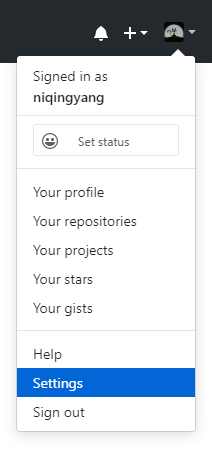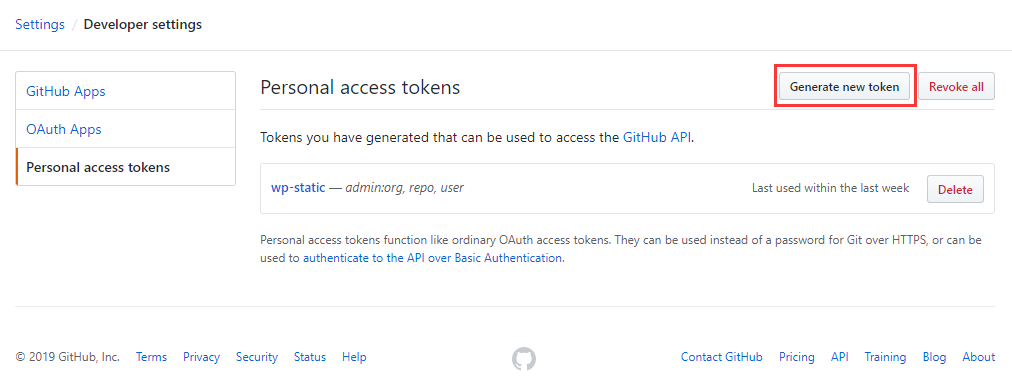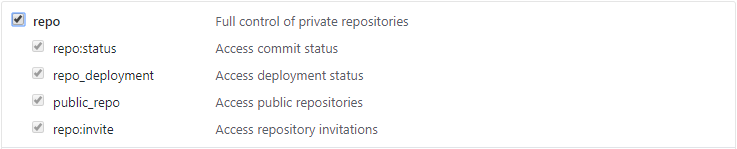1. Github 账户名仓库名access token 粘帖到该插件设置页面中相应的位置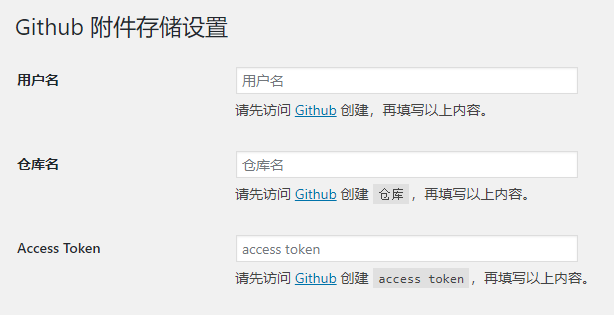1. URL前缀的格式为 https://raw.githubusercontent.com/{用户名}/{仓库名}/master/，或者 https://raw.githubusercontent.com/{用户名}/{仓库名}/master/{本地文件夹}，“本地文件夹” 务必与上面设置的 “本地文件夹” 保持一致（结尾无/）

2. 设置自己的域名：可以使用 Github Page 的域名，也可以用 CNAME 将自己的域名解析到 Github Page 的域名 上, 然后将https://raw.githubusercontent.com/{用户名}/{仓库名}/master/ 替换成自己的域名就可以了

3. 💥💥 国内访问 github 速度不是很理想，可以使用 jsdelivr 免费加速 github，只需要将 URL 前缀设置格式为 https://cdn.jsdelivr.net/gh/{用户名}/{仓库名}/即可

## 插件预览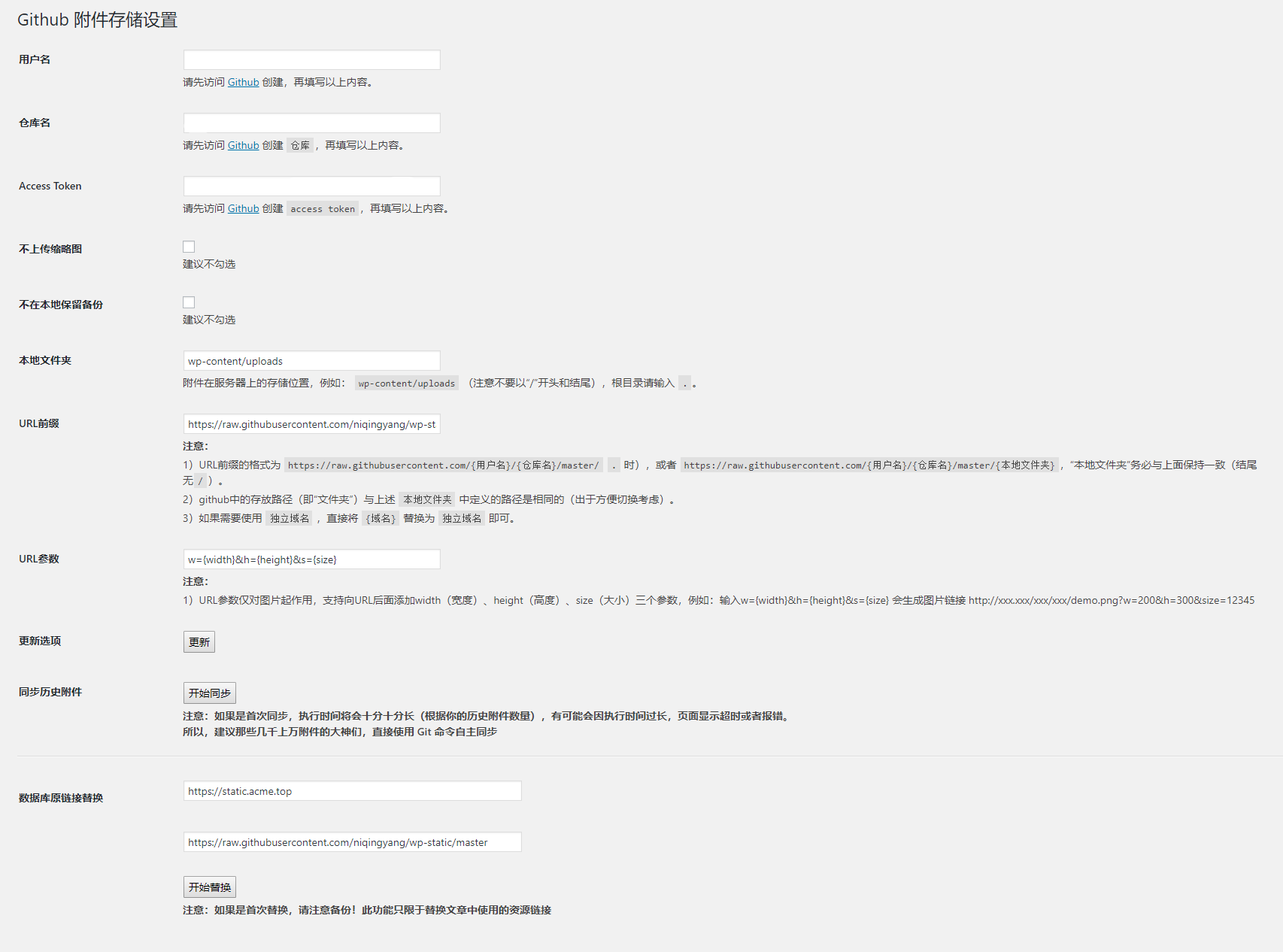## 常见问题

1、怎么替换文章中之前的旧资源地址链接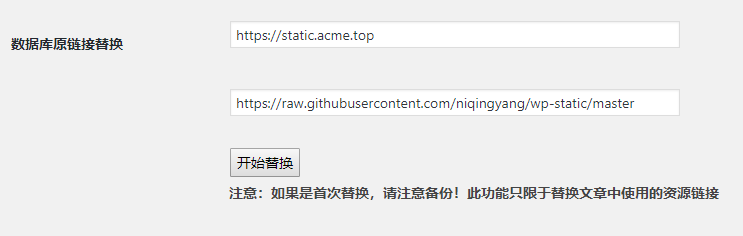## WP-Editor.md

/**
* 文章编辑页面从媒体选择器中插入图片
*
* @param string $html * @param integer$id
* @param string $caption * @param string$title
* @param string align * @param stringurl
* @param string $size * @param string$alt
* @return mixed
*/
function acme_image_send_to_editor ($html,$id, $caption,$title, align,url, $size,$alt)
{
$attrs = wp_kses_hair($html, wp_allowed_protocols());

$attrs = array_column($attrs, 'value', 'name');

$src =$url . '?w=' . $attrs['width'] . '&h=' .$attrs['height'] . '&id=' . $id;$html = str_replace($url,$src, $html); return$html;
}

/**
* 粘帖图片上传，替换EditorMD插件的粘帖上传
*/
function acme_imagepaste_action_callback ()
{
$result = array( 'error' => '' );$upload = wp_upload_dir();
$uploadUrl =$upload['url'];
$uploadDir =$upload['path'];
$extension = ''; list($data, $image) = explode(';',$_REQUEST['dataurl']);
list($field,$type) = explode(':', $data); list($encoding, $content) = explode(',',$image);
if($type == 'image/png') {$extension = 'png';
}
$name = md5($_REQUEST['dataurl']);
if(! $extension) {$result['error'] = "Could not determine image extension type";
}
else
{
$file =$uploadDir . '/paste-' . $name . '.' .$extension;
file_put_contents($file, base64_decode($content));
// 获取图片尺寸
$size = @getimagesize($file);

if($size == false) {$result['error'] = "Could not get image size";
}
else
{
$_FILES['file'] = [ 'name' => '/paste-' .$name . '.' . $extension, 'type' => 'image/' .$extension,
'tmp_name' => $file, 'error' => 0, 'size' => filesize($file)
];

$time = current_time('mysql');$overrides = array(
'test_form' => false,
'action' => 'acme_imagepaste_action'
);

$file = wp_handle_upload($_FILES['file'], $overrides,$time);

if(isset($file['error'])) {$result['error'] = $file['error']; } else { if(strpos($file['url'], '?'))
{
$url =$file['url'];
}
else
{
$url =$file['url'] . '?w=' . $size . '&h=' .$size;
}

$result['w3tc'] = 0;$result['url'] = $url;$result['elementid'] = $_REQUEST['elementid']; } } } echo json_encode($result);
die();
}



## 更新记录

V1.0 - 2019/07/23

• 使用 GitHub 仓库存储 WordPress 站点图片等多媒体文件
• 可配置是否上传缩略图和是否保留本地备份
• 本地删除可同步删除腾讯云上面的文件
• 支持替换数据库中旧的资源链接地址
• 支持在图片链接地址后面自定义拼接图片宽度、高度、大小三个参数

V1.1 - 2020/06/28

• 根据 Github API 的变更，将 access_token 参数淘汰，请求时将其放入请求头中
• 优化上传，偶发性的会发生 cURL error 35 错误，这种错误会重新尝试最多三次上传
• 优化上传，发生未知的错误会将其捕获并反馈到前端显示上传失败
• 为便于调试上传，支持将上传及错误信息记录日志，默认是关闭的